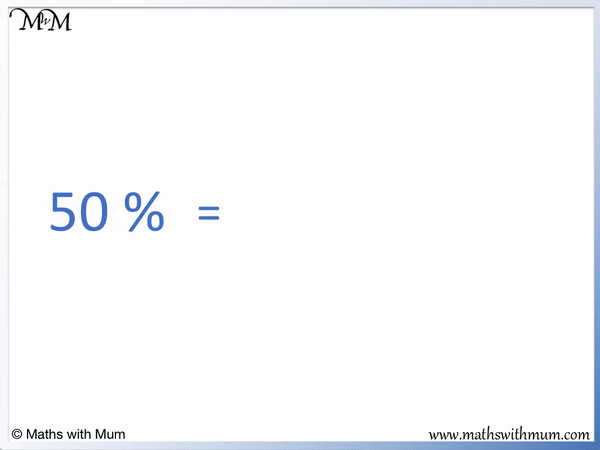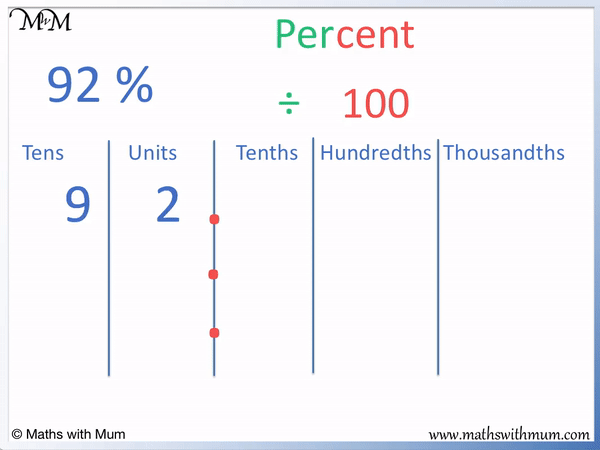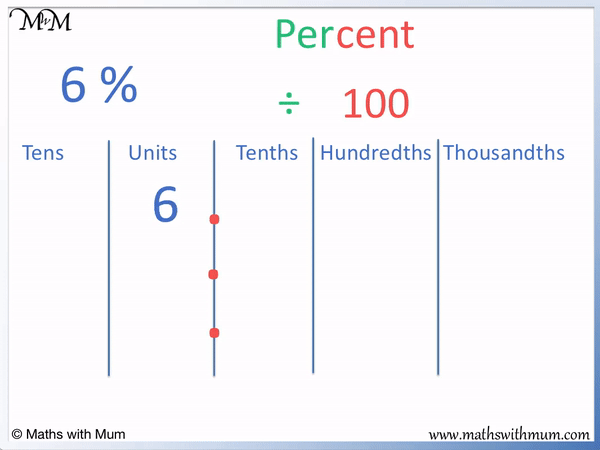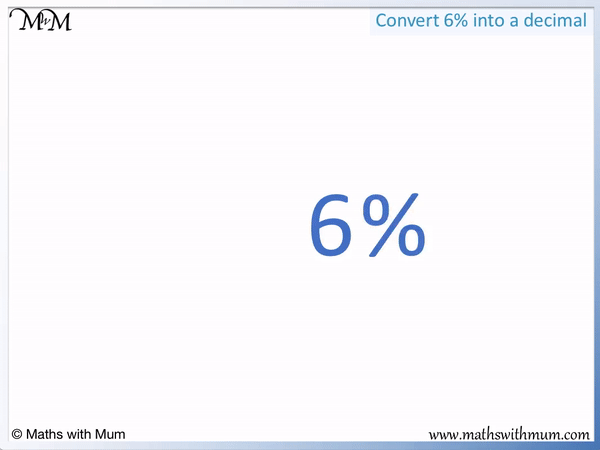# How to Write Percentages as Decimals

How to Write Percentages as Decimals• The percent symbol is %.
• Per means ‘divide by’ and cent means ‘100’.
• To convert from a percent to a decimal, we divide the number by 100.
• 50 divided by 100 is 0.5.
• 50% written as a decimal number is 0.5.
• We do not write the percentage sign after our answer now that we have divided by 100.
• 50% is the same as one half. 0.5 is how we write one half as a decimal.
To write a percent as a decimal, remove the % sign and divide by 100.• To write 92% as a decimal, we remove the % sign and divide it by 100.
• Removing the percentage sign, we have 92.
• To divide by 100, simply move the decimal point from the end of the number to the left by 2 places.
• The decimal point moves from 92. to .92 when we divide by 100.
• We write a zero in front of the decimal point to get 0.92.
• 92% written as a decimal is 0.92.Supporting Lessons# Writing Percentages as Decimals

## How to Convert a Percent to a Decimal

To convert a percent to a decimal, remove the percentage symbol and divide the number by 100. To divide by 100, move the decimal point 2 places to the left. For example, 92% as a decimal is 0.92.

Percent means to divide by 100.

This is because the word ‘per’ can mean ‘divide by’ and the word ‘cent’ means ‘100’. The percent symbol ‘%’ can mean ‘divide by 100’.

Here is an example of writing a percentage in decimal form. 92%, can be read to mean 92 ‘divided by 100’.

To divide a number by 100, we divide by 10 and then divide by 10 again.When a number is divided by 10, the digits move one place value column to the right.

92 ÷ 10 = 9.2. We divide by 10 again.

9.2 ÷ 10 = 0.92. We write the 0 in front of the decimal point because there are no more digits. There is not a digit to put in the ones column, so we put a 0 to show this.

Dividing a number by 100 is the same as dividing it by 10 and then by 10 again.

When dividing a number by 100, the digits each moved 2 places right.

Rather than moving each of the digits, it is easier to move the decimal point the other way instead.

To divide by 100, simply move the decimal point to the left two places.When teaching converting percents to decimals, it is easier to divide by 100 by moving the decimal point two places left instead of moving all of the digits two places right. Students are less likely to make a mistake if they only have the decimal point to move rather than every single digit. We can leave all the digits where they are and just focus on carefully moving the decimal point two places left.

92% written in decimal form is 0.92.

Here is another example of writing a percent in decimal form.

We will write 6% as a decimal number by removing the % sign and dividing by 100.

To divide by 100, move each digit two places to the right.6 ÷ 10 = 0.6 and then 0.6 ÷ 10 = 0.06.

6 ÷ 100 = 0.06 and so, 6% as a decimal is 0.06.

Alternatively, we can divide 6 by 100 by moving the decimal point two places left.The decimal point must move twice.

We move the decimal point once to go from 6. to .6 and now we need to move it once more.

We need to put a 0 in front of the 6 digit so that we can jump over that.

We go from 0.6 to .06 and we write a zero in front of the decimal point to get 0.06.

6 ÷ 100 = 0.06 and so 6% as a decimal is 0.06.

It is important to move the decimal point carefully when converting a percentage to a decimal.

It is a common mistake to write percentages such as 6% as 0.6 instead of 0.06. It is common to miss out the extra 0 after the decimal point.

When converting a single digit percentage to a decimal, there will always be a 0 between the decimal point and the digit. In general a% can be written as a decimal as 0.0a.

For example, 5% is 0.05, 3% is 0.03 and 9% is 0.09.

To converting a double digit percentage to a decimal, simply write the two digits immediately after the decimal point. In general ab% can be written as a decimal as 0.ab.

For example, 25% is 0.25, 37% is 0.37 and 99% is 0.99.

## Converting a Decimal Percentage to a Decimal

No matter what the percentage is, divide it by 100 to write it as a decimal number. If the percentage is already a decimal number, then when it is written as a decimal, it will be 100 times smaller. For example 3.2% as a decimal is 0.032.

It is possible to have decimal percentages. For example 3.2%.

The rule remains the same. Remove the % sign and divide the number by 100.To divide by 100, we divide by 10 twice.

3.2 ÷ 10 = 0.32 and then 0.32 ÷ 10 = 0.032.

3.2% is 0.032 as a decimal. Because the % sign has been removed, the number has now got 100 times smaller.

It can be easier to move the decimal point two places to the left.Moving the decimal point two places we go from 3.2 to 0.32 to 0.032.

3.2% is 0.032.

Here are some examples of converting percentages to decimals.

Percentage Decimal
48% 0.48
2% 0.02
0.6% 0.006
2.57% 0.0257
200% 2
354% 3.54

Simply divide the percentage by 100 to get it in decimal form.Now try our lesson on Converting Decimals to Percentages where we learn how to write a decimal as a percentage.